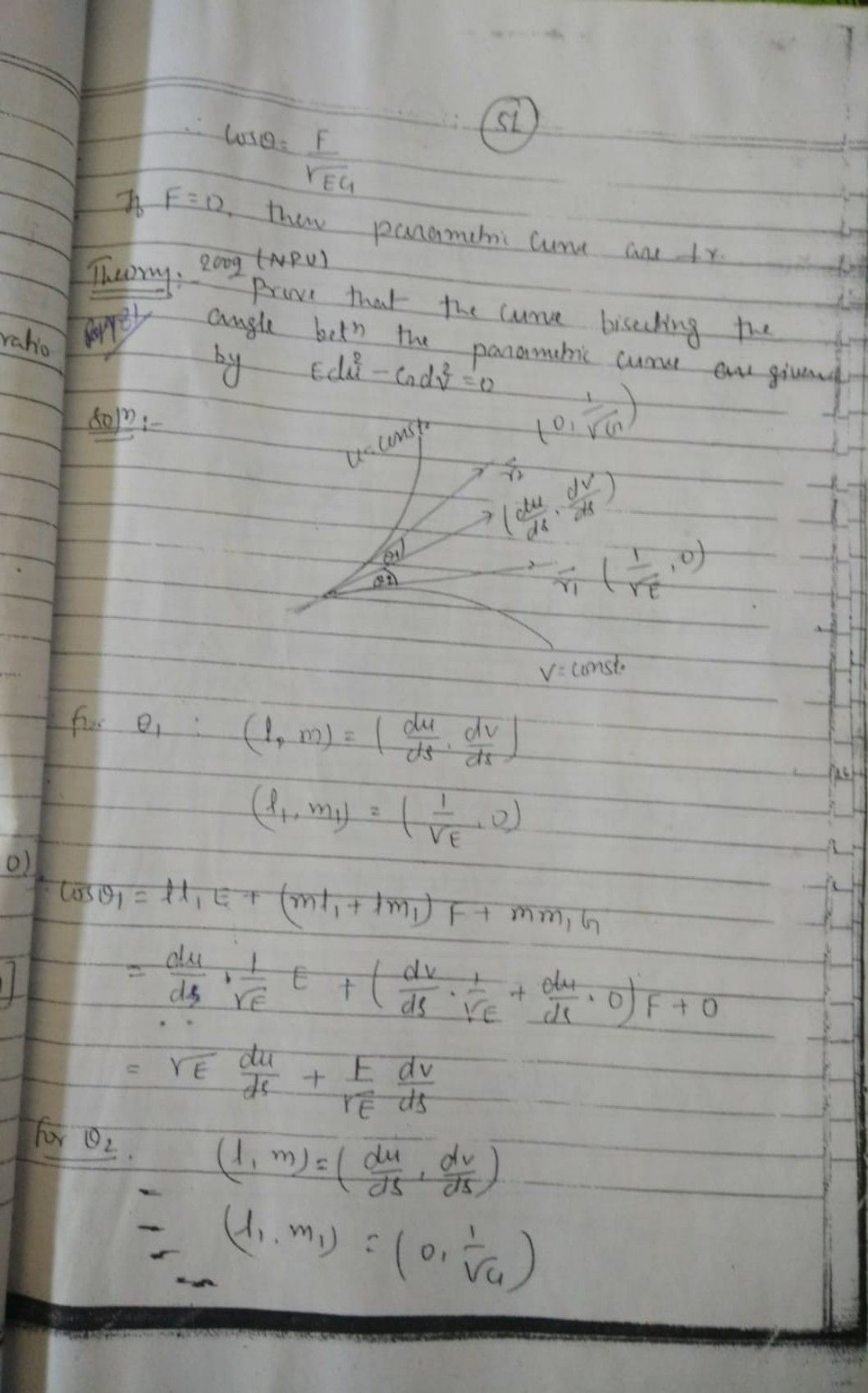Symbol
Problem$β$ A beverage company that distributes cases of water claims the weights of the cases are normally distributed with a mean of $500$ ounces. While trying not to exceed the weight limit allowed on a transport truck, Phil, the manager, begins to calculate which interval of weights would contain the largest proportion of water. He decides that the interval $1$ $490oz$ $to51002$ would contain a larger proportion than $5100z$ to $5300z$ His coworker disagrees. Is Phil or his coworker correct? Justify your answer.
1st-6th grade
Probability and Statistics
SolutionQanda teacher - Chandrabha